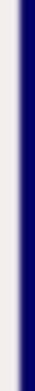homework help forum today's math problem links utilities previous math problem feedback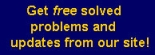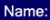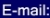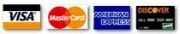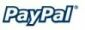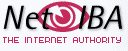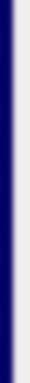# MATH HOMEWORK HELP

Problem: Winter wheat, while finding the amount of seed needed to plant his three square wheat fields. Hank observed that the side of one field was 1 kilometer longer than the size of the smallest field and that the side of the largest field was 3 kilometers longer than the side of the smallest field. If the total area of the three is 38 square kilometers, then what is the area of each field?

Solution: Let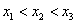be the sides of the fields. We know that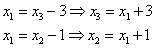The total area is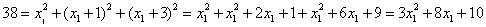This implies that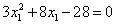which has two solutions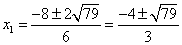but one of the solutions is negative, so we disregard it. Therefore,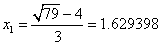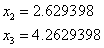MGT - Your source of Math and Statistics Homework Help